# Class 7 Maths NCERT Solutions for Chapter – 9 Rational Numbers Ex – 9.1

## Rational Numbers

Question 1.
List five rational numbers between
(i)
-1 and 0
(ii) -2 and -1
(iii)$\frac { -4 }{ 5 }$ and$\frac { -2 }{ 3 }$
(iv) –$\frac { 1 }{ 2 }$ and$\frac { 2 }{ 3 }$

Solution:

Question 2.
Write four more rational numbers in each of the following patterns :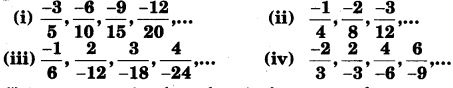Solution:

Question 3.
Give four rational numbers equivalent to
(i)$\frac { -2 }{ 7 }$
(ii)$\frac { 5 }{ -3 }$
(iii)$\frac { 4 }{ 9 }$

Solution:

Question 4.
Draw the number line and represent the following rational numbers on it :
(i)$\frac { 3 }{ 4 }$
(ii)$\frac { -5 }{ 8 }$
(iii)$\frac { -7 }{ 4 }$
(iv)$\frac { 7 }{ 8 }$

Solution:
(i) In order to represent$\frac { 3 }{ 4 }$ on the number line, we first draw a number line and mark a point O on it to represent zero. Now, mark the point P representing 3 on the number line as shown. Now, divide the segment OP into four equal parts. Let A, B, C be the points of division so that OA = AB =BC = CP. By construction, OA is three- fourth of OP. So, A represents the rational number$\frac { 3 }{ 4 }$(ii) In order to represent$\frac { -5 }{ 8 }$ on the number line, we first draw a number line and mark a point O on it to represent zero. Now, mark the point P representing -5 on it as shown. Now, divide the segment OP into eight equal parts. Let A, B, C, D, E, F, G be the points of division such that OA = AB = BC = CD = DE = EF = FG = GP. By construction, OA is one-eighth of OP. So, A represents the rational number$\frac { -5 }{ 8 }$.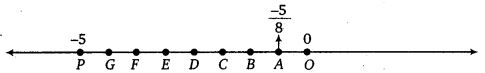(iii) In order to represent$\frac { -7 }{ 4 }$ on the number line, we first draw a number line and mark a point O on it to represent zero. Now, mark a point P to represent -7 on the number line. Now,divide the segment OP into 4 equal parts. Let A, B, C be the points of division so that OA = AB = BC = CP. By construction, OA is one-fourth of OP. Therefore, A represents the rational number$\frac { -7 }{ 4 }$.(iv) In order to represent$\frac { 7 }{ 8 }$ on the number line, we first draw a number line and mark a point O on it to represent zero. Now, mark the point P to represent 7 on the number line. Now, divide the segment OP into 8 equal parts. Let A, B, C, D, E, F, G be the points of division such that OA = AB = BC = CD = DE = EF = FG = GP. By construction, OA is one-eighth of OP. Therefore, A represents the rational number$\frac { 7 }{ 8 }$.Question 5.
The points P, Q, R, S, T, U, A and B on the number line are such that, TR = RS = SU and AP = PQ = QB. Name the rational numbers represented by P, Q, R and S.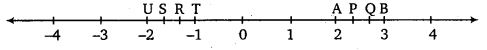Solution:

Question 6.
Which of the following pairs represents the same rational number ?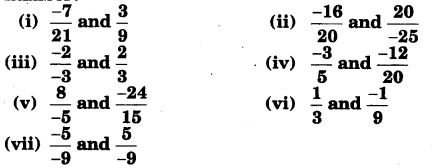Solution:

Question 7.
Rewrite the following rational numbers in the simplest form :
(i)$\frac { -8 }{ 6 }$
(ii)$\frac { 25 }{ 45 }$
(iii)$\frac { -44 }{ 72 }$
(iv)$\frac { -8 }{ 10 }$
Solution:

Question 8.
Fill in the boxes with the correct symbol out of >, <, and =.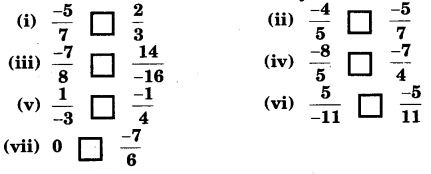Solution:
(i) Clearly,$\frac { -5 }{ 7 }$ is a negative rational number and$\frac { 2 }{ 3 }$ is a positive rational number. We know that every negative rational number is less than every positive rational number. Therefore,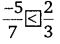(ii) Clearly, denominators of the given rational numbers are positive. The denominators are 5 and 7. Their L.C.M. is 35. So, we first express each rational number with 35 as common denominator.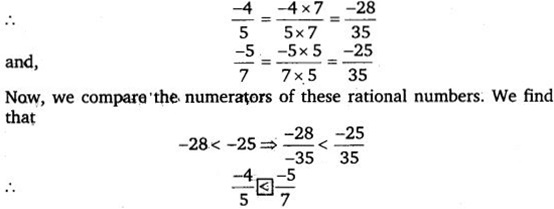(iii) First- we write each one of the given rational numbers with positive denominator :

Clearly, denominator of$\frac { -7 }{ 8 }$ is positive. The denominator of$\frac { 14 }{ -16 }$ is negative. So, we express it with positive denominator as follows :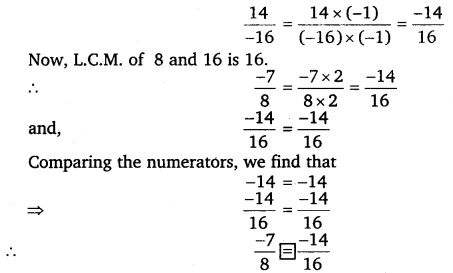(iv) Clearly, the denominators of the given rational numbers are positive. The denominators are 5 and 4.
Their L.C.M. is 20. So, we first express each rational number with 20 as common denominator.(v) First we write each one of the given rational numbers with positive denominator.

Clearly, denominator of$\frac { 1 }{ -3 }$ is negative. So, expressing it with positive denominator as follows :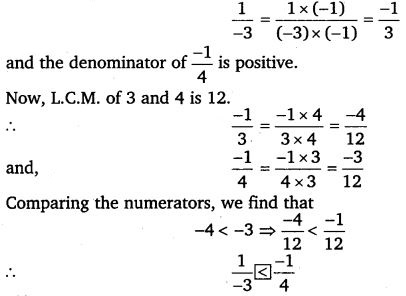(vi) First we write each one of the given rational numbers with positive denominator.(vii) Since every negative rational number is less than 0,Question 9.
Which is greater in each of the following :
(i)$\frac { 2 }{ 3 }$,$\frac { 5 }{ 2 }$
(ii)$\frac { -5 }{ 6 }$,$\frac { -4 }{ 3 }$
(iii)$\frac { -3 }{ 4 }$,$\frac { 2 }{ -3 }$
(iv)$\frac { -1 }{ 4 }$,$\frac { 1 }{ 4 }$
(v) -3$\frac { 2 }{ 7 }$,-3$\frac { 4 }{ 5 }$

Solution:

Question 10.
Write the following rational numbers in ascending order :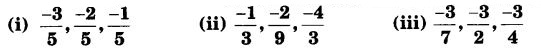Solution: